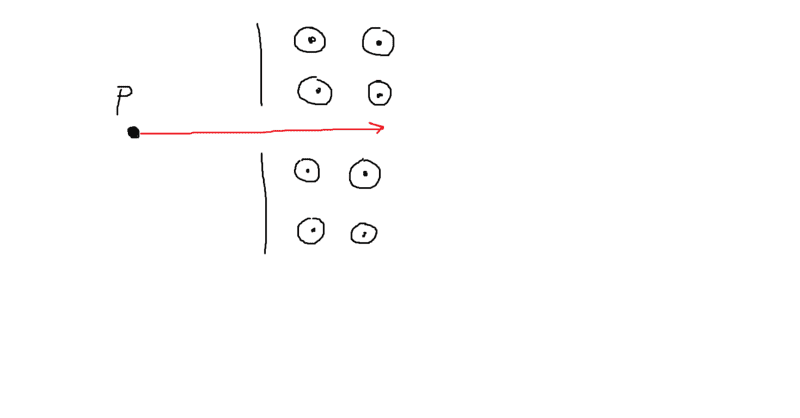# Is today's exam question unsolvable? - Proton Motion in a Magnetic Field

• APUGYael
In summary, a member of the forum had an electromagnetic exam and encountered a problem that seemed unsolvable due to insufficient information. The problem involved calculating the total distance traveled by a proton in a magnetic field with given variables and a figure. The moderator suggested that additional information was needed to determine the starting and ending conditions. The member's professor later clarified that the proton would travel half a circle before hitting the wall, but the figure provided was unclear and could have been interpreted differently. Some members of the forum also shared their assumptions and criticisms of the question. Overall, the lack of clarity in the question was noted and it was suggested that question setters should review their questions before using them in an exam.

#### APUGYael

<Moderator's note: Moved from a technical forum and thus no template.>

Hello all,

Earlier today I had an electromagnetism exam, and it seems to me that this problem is unsolvable.
There's just too little information given.

A proton enters an uniform magnetic field. It's initial speed v is perpendicular to the magnetic field.
The strength of the magnetic field is B. You also know the direction of the magnetic field (see: https://imgur.com/a/HSTT7Qh).Calculate the total distance the proton travels.

So let's say that, because it's initial speed is perpendicular, the force acting on the proton equals:
F = qvB
q = charge
v = speed
B = magnetic field strength

This force is the centripetal force:
qvB = pv/r

p = momentum (mv)

So.. Let's solve for r.
r = p/qB

But that doesn't help much.

See: you don't know how long the proton is present in the magnetic field. You could calculate the circumference of a circle with radius r, you could calculate the time it takes the proton to travel 2πr but you still wouldn't be able to calculate the total distance traveled without a given time.

Anyway, my girlfriend is convinced this is solvable. Am I overlooking something?

#### Attachments

Last edited by a moderator:
APUGYael said:
Calculate the total distance the proton travels.
You need some additional qualifying information that determines what the start and end conditions are, i.e., what is the distance traveled by the proton between A and B, where A and B are somehow identified. Without access to your exam, it is impossible to say whether or not this information was suitably identifiable, e.g., through a figure.

Orodruin said:
You need some additional qualifying information that determines what the start and end conditions are, i.e., what is the distance traveled by the proton between A and B, where A and B are somehow identified. Without access to your exam, it is impossible to say whether or not this information was suitably identifiable, e.g., through a figure.

The drawn figure is the exact figure given but drawn on paint. Nothing was left out. Same for the given variables and description of the problem.

There are many assumptions you can make. One that would be reasonable would be that, from what can be seen from the figure, the magnetic field starts abruptly and the proton enters perpendicular to the zone where the field is non-zero. The question could intend to ask for the distance traveled in the magnetic field. However, based on the information you have given here, any such guess is just conjecture.

Orodruin said:
There are many assumptions you can make. One that would be reasonable would be that, from what can be seen from the figure, the magnetic field starts abruptly and the proton enters perpendicular to the zone where the field is non-zero. The question could intend to ask for the distance traveled in the magnetic field. However, based on the information you have given here, any such guess is just conjecture.

I just got a reply from my professor.
He told me I should've made the assumption that the well extends and such that the distance the proton travels is half of a circle before hitting the wall. To me the figure seems flawed: the figure should've been clarified or more information should've been given, although I do see his point.

APUGYael said:
He told me I should've made the assumption that the well extends and such that the distance the proton travels is half of a circle before hitting the wall.
As stated above, this is what I would have assumed, but I agree it could be stated clearer.

I didn’t even realize that vertical line was supposed to represent a wall. I thought it was just the edge of the region with the field. I would have said that it goes 1/2 circle around and then continues straight on forever (neglecting gravity or other fields)

If it was supposed to be an exam question then it is a very poor one. Why don't question setters read what they have written and see if it actually means what they want it to mean?
I think @Dale 's interpretation is favourite but why isn't it made clear? Perhaps it's part Parapsychology Exam.